# 4 digit Addition without Regrouping

4 Digit Addition without Regrouping – Question 1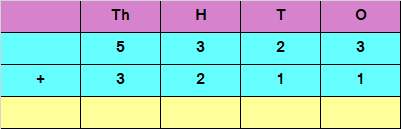Explanation

Adding the digits at ones place,
3 + 1 = 4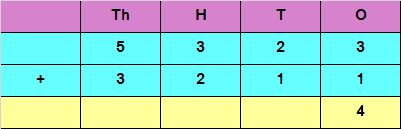Adding the digits at tens place,
2 + 1 = 3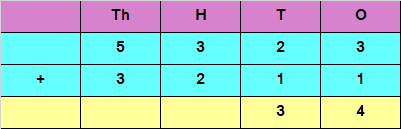Adding the digits at hundreds place,
3 + 2 = 5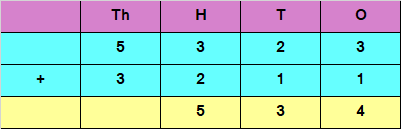Adding the digits at thousands place,
5 + 3 = 8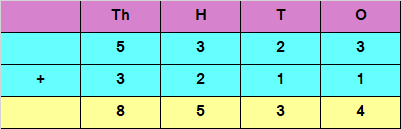Hence, the sum of the given numbers is 8534

4 Digit Addition without Regrouping – Question 2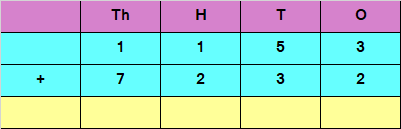Explanation

Adding the digits at ones place,
3 + 2 = 5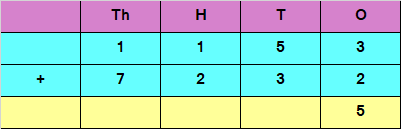Adding the digits at tens place,
5 + 3 = 8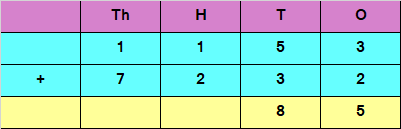Adding the digits at hundreds place,
1 + 2 = 3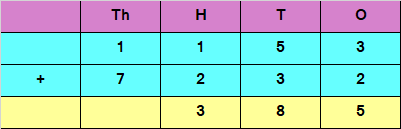Adding the digits at thousands place,
1 + 7 = 8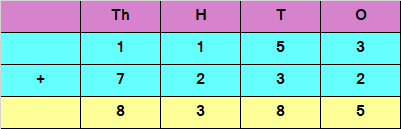Hence, the sum of the given numbers is 8385